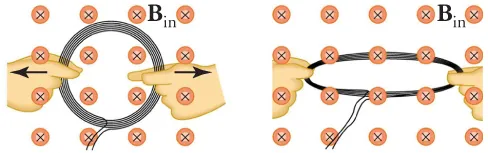Physics

# Concept Items

PhysicsConcept Items

### Concept Items

#### 20.1Magnetic Fields, Field Lines, and Force

1 .
If you place a small needle between the north poles of two bar magnets, will the needle become magnetized?
1. Yes, the magnetic fields from the two north poles will point in the same directions.
2. Yes, the magnetic fields from the two north poles will point in opposite directions.
3. No, the magnetic fields from the two north poles will point in opposite directions.
4. No, the magnetic fields from the two north poles will point in the same directions.
2.

If you place a compass at the three points in the figure, at which point will the needle experience the greatest torque? Why?1. The density of the magnetic field is minimized at B, so the magnetic compass needle will experience the greatest torque at B.
2. The density of the magnetic field is minimized at C, so the magnetic compass needle will experience the greatest torque at C.
3. The density of the magnetic field is maximized at B, so the magnetic compass needle will experience the greatest torque at B.
4. The density of the magnetic field is maximized at A, so the magnetic compass needle will experience the greatest torque at A.
3 .
In which direction do the magnetic field lines point outside the south pole of a magnet?
1. Outside the magnet the direction of magnetic field lines is towards the south pole of the magnet.
2. Outside the magnet the direction of magnetic field lines is away from the south pole of the magnet.

#### 20.2Motors, Generators, and Transformers

4 .
Consider the angle between the area vector and the magnetic field in an electric motor. At what angles is the torque on the wire loop the greatest?
1. $0^\circ$and $180^\circ$
2. $45^\circ$ and $135^\circ$
3. $90^\circ$ and $270^\circ$
4. $225^\circ$ and $315^\circ$
5.

What is a voltage transformer?

1. A transformer is a device that transforms current to voltage.
2. A transformer is a device that transforms voltages from one value to another.
3. A transformer is a device that transforms resistance of wire to voltage.
6.

Why is electric power transmitted at high voltage?

1. To increase the current for the transmission
2. To reduce energy loss during transmission
3. To increase resistance during transmission
4. To reduce resistance during transmission

#### 20.3Electromagnetic Induction

7.

Yes or no—Is an emf induced in the coil shown when it is stretched? If so, state why and give the direction of the induced current.1. No, because induced current does not depend upon the area of the coil.
2. Yes, because area of the coil decreases; the direction of the induced current is counterclockwise.
3. Yes, because area of the coil decreases; the direction of the induced current is clockwise.
4. Yes, because the area of the coil does not change; the direction of the induced current is clockwise.
8 .
What is Lenz’s law?
1. If induced current flows, its direction is such that it adds to the changes which induced it.
2. If induced current flows, its direction is such that it opposes the changes which induced it.
3. If induced current flows, its direction is always clockwise to the changes which induced it.
4. If induced current flows, its direction is always counterclockwise to the changes which induced it.
9.

Explain how magnetic flux can be zero when the magnetic field is not zero.

1. If angle between magnetic field and area vector is 0°, then its sine is also zero, which means that there is zero flux.
2. If angle between magnetic field and area vector is 45°, then its sine is also zero, which means that there is zero flux.
3. If angle between magnetic field and area vector is 60°, then its cosine is also zero, which means that there is zero flux.
4. If the angle between magnetic field and area vector is 90°, then its cosine is also zero, which means that there is zero flux.
Order a print copy

As an Amazon Associate we earn from qualifying purchases.